## Convolution Theorem

Letandbe arbitrary functions of timewith Fourier Transforms. Take(1)(2)

wheredenotes the inverse Fourier Transform (where the transform pair is defined to have constants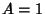and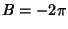). Then the Convolution is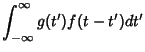(3)

Interchange the order of integration,(4)

So, applying a Fourier Transform to each side, we have(5)

The convolution theorem also takes the alternate forms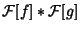(6)(7)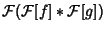(8)

Arfken, G. Convolution Theorem.'' §15.5 in Mathematical Methods for Physicists, 3rd ed. Orlando, FL: Academic Press, pp. 810-814, 1985.
Bracewell, R. Convolution Theorem.'' The Fourier Transform and Its Applications. New York: McGraw-Hill, pp. 108-112, 1965.